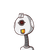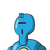# write an general form of an quadratic equation and writes the nature of the roots of quadratic equation​

write an general form of an quadratic equation and writes the nature of the roots of quadratic equation​

### 2 thoughts on “write an general form of an quadratic equation and writes the nature of the roots of quadratic equation​”

1.Step-by-step explanation:

ax²+bx+c=0

a is not equal to zero

Nature of the roots –

b² -4ac=0 (Two equal and real roots)

b² -4ac>0 (Two distinct and real roots)

b²-4ac<0 (No real roots)

2.